1 Sep 2018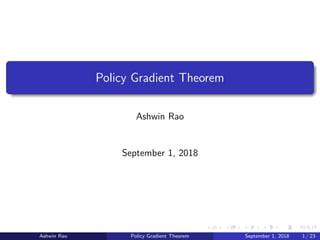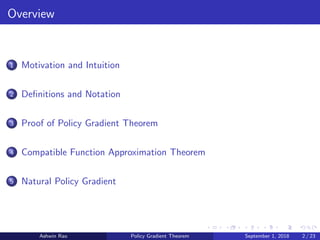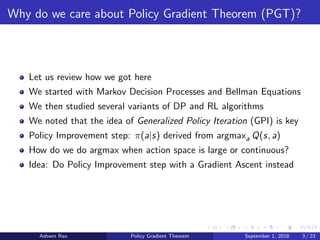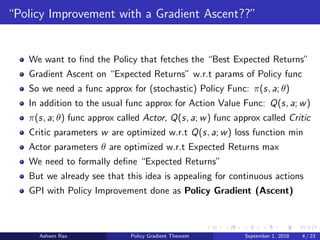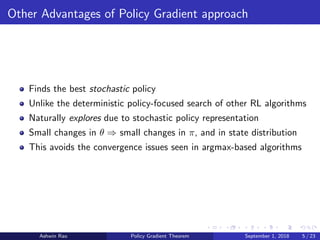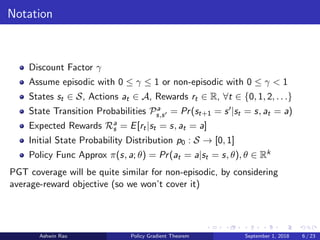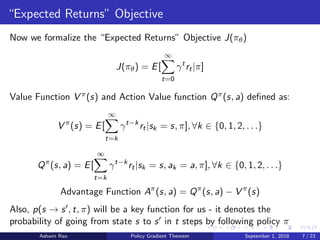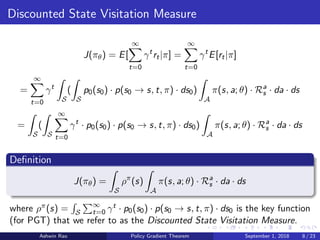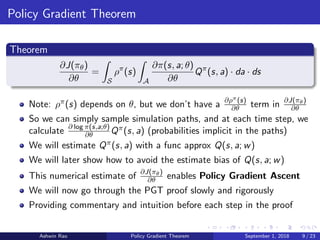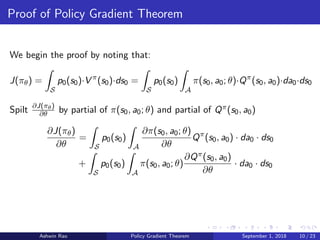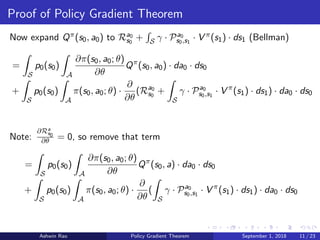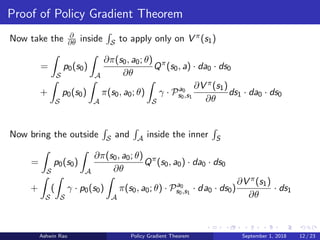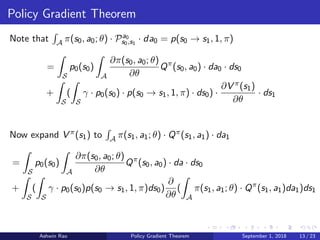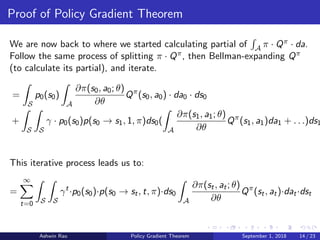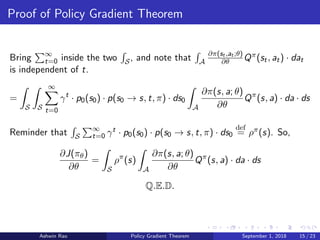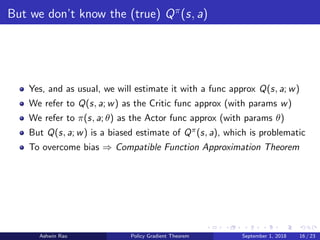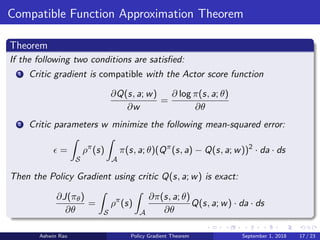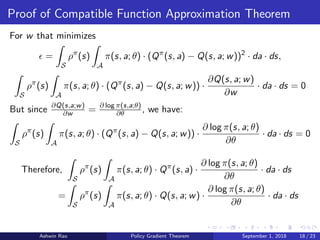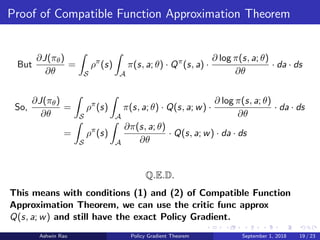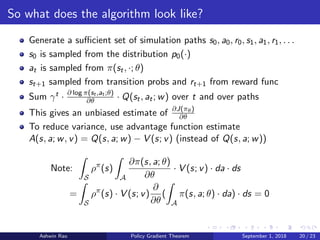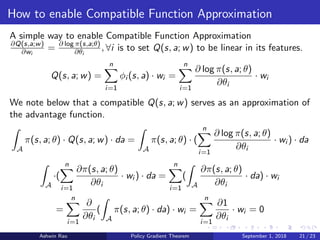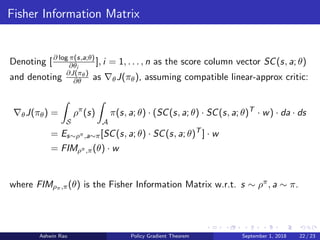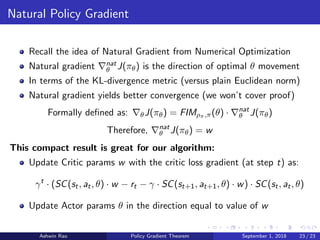1 sur 23

• 1. Policy Gradient Theorem Ashwin Rao September 1, 2018 Ashwin Rao Policy Gradient Theorem September 1, 2018 1 / 23
• 2. Overview 1 Motivation and Intuition 2 Deﬁnitions and Notation 3 Proof of Policy Gradient Theorem 4 Compatible Function Approximation Theorem 5 Natural Policy Gradient Ashwin Rao Policy Gradient Theorem September 1, 2018 2 / 23
• 3. Why do we care about Policy Gradient Theorem (PGT)? Let us review how we got here We started with Markov Decision Processes and Bellman Equations We then studied several variants of DP and RL algorithms We noted that the idea of Generalized Policy Iteration (GPI) is key Policy Improvement step: π(a|s) derived from argmaxa Q(s, a) How do we do argmax when action space is large or continuous? Idea: Do Policy Improvement step with a Gradient Ascent instead Ashwin Rao Policy Gradient Theorem September 1, 2018 3 / 23
• 4. “Policy Improvement with a Gradient Ascent??” We want to ﬁnd the Policy that fetches the “Best Expected Returns” Gradient Ascent on “Expected Returns” w.r.t params of Policy func So we need a func approx for (stochastic) Policy Func: π(s, a; θ) In addition to the usual func approx for Action Value Func: Q(s, a; w) π(s, a; θ) func approx called Actor, Q(s, a; w) func approx called Critic Critic parameters w are optimized w.r.t Q(s, a; w) loss function min Actor parameters θ are optimized w.r.t Expected Returns max We need to formally deﬁne “Expected Returns” But we already see that this idea is appealing for continuous actions GPI with Policy Improvement done as Policy Gradient (Ascent) Ashwin Rao Policy Gradient Theorem September 1, 2018 4 / 23
• 5. Other Advantages of Policy Gradient approach Finds the best stochastic policy Unlike the deterministic policy-focused search of other RL algorithms Naturally explores due to stochastic policy representation Small changes in θ ⇒ small changes in π, and in state distribution This avoids the convergence issues seen in argmax-based algorithms Ashwin Rao Policy Gradient Theorem September 1, 2018 5 / 23
• 6. Notation Discount Factor γ Assume episodic with 0 ≤ γ ≤ 1 or non-episodic with 0 ≤ γ < 1 States st ∈ S, Actions at ∈ A, Rewards rt ∈ R, ∀t ∈ {0, 1, 2, . . .} State Transition Probabilities Pa s,s = Pr(st+1 = s |st = s, at = a) Expected Rewards Ra s = E[rt|st = s, at = a] Initial State Probability Distribution p0 : S → [0, 1] Policy Func Approx π(s, a; θ) = Pr(at = a|st = s, θ), θ ∈ Rk PGT coverage will be quite similar for non-episodic, by considering average-reward objective (so we won’t cover it) Ashwin Rao Policy Gradient Theorem September 1, 2018 6 / 23
• 7. “Expected Returns” Objective Now we formalize the “Expected Returns” Objective J(πθ) J(πθ) = E[ ∞ t=0 γt rt|π] Value Function V π(s) and Action Value function Qπ(s, a) deﬁned as: V π (s) = E[ ∞ t=k γt−k rt|sk = s, π], ∀k ∈ {0, 1, 2, . . .} Qπ (s, a) = E[ ∞ t=k γt−k rt|sk = s, ak = a, π], ∀k ∈ {0, 1, 2, . . .} Advantage Function Aπ (s, a) = Qπ (s, a) − V π (s) Also, p(s → s , t, π) will be a key function for us - it denotes the probability of going from state s to s in t steps by following policy π Ashwin Rao Policy Gradient Theorem September 1, 2018 7 / 23
• 8. Discounted State Visitation Measure J(πθ) = E[ ∞ t=0 γt rt|π] = ∞ t=0 γt E[rt|π] = ∞ t=0 γt S ( S p0(s0) · p(s0 → s, t, π) · ds0) A π(s, a; θ) · Ra s · da · ds = S ( S ∞ t=0 γt · p0(s0) · p(s0 → s, t, π) · ds0) A π(s, a; θ) · Ra s · da · ds Deﬁnition J(πθ) = S ρπ (s) A π(s, a; θ) · Ra s · da · ds where ρπ(s) = S ∞ t=0 γt · p0(s0) · p(s0 → s, t, π) · ds0 is the key function (for PGT) that we refer to as the Discounted State Visitation Measure. Ashwin Rao Policy Gradient Theorem September 1, 2018 8 / 23
• 9. Policy Gradient Theorem Theorem ∂J(πθ) ∂θ = S ρπ (s) A ∂π(s, a; θ) ∂θ Qπ (s, a) · da · ds Note: ρπ(s) depends on θ, but we don’t have a ∂ρπ(s) ∂θ term in ∂J(πθ) ∂θ So we can simply sample simulation paths, and at each time step, we calculate ∂ log π(s,a;θ) ∂θ Qπ(s, a) (probabilities implicit in the paths) We will estimate Qπ(s, a) with a func approx Q(s, a; w) We will later show how to avoid the estimate bias of Q(s, a; w) This numerical estimate of ∂J(πθ) ∂θ enables Policy Gradient Ascent We will now go through the PGT proof slowly and rigorously Providing commentary and intuition before each step in the proof Ashwin Rao Policy Gradient Theorem September 1, 2018 9 / 23
• 10. Proof of Policy Gradient Theorem We begin the proof by noting that: J(πθ) = S p0(s0)·V π (s0)·ds0 = S p0(s0) A π(s0, a0; θ)·Qπ (s0, a0)·da0·ds0 Spilt ∂J(πθ) ∂θ by partial of π(s0, a0; θ) and partial of Qπ(s0, a0) ∂J(πθ) ∂θ = S p0(s0) A ∂π(s0, a0; θ) ∂θ Qπ (s0, a0) · da0 · ds0 + S p0(s0) A π(s0, a0; θ) ∂Qπ(s0, a0) ∂θ · da0 · ds0 Ashwin Rao Policy Gradient Theorem September 1, 2018 10 / 23
• 11. Proof of Policy Gradient Theorem Now expand Qπ(s0, a0) to Ra0 s0 + S γ · Pa0 s0,s1 · V π(s1) · ds1 (Bellman) = S p0(s0) A ∂π(s0, a0; θ) ∂θ Qπ (s0, a0) · da0 · ds0 + S p0(s0) A π(s0, a0; θ) · ∂ ∂θ (Ra0 s0 + S γ · Pa0 s0,s1 · V π (s1) · ds1) · da0 · ds0 Note: ∂Ra s0 ∂θ = 0, so remove that term = S p0(s0) A ∂π(s0, a0; θ) ∂θ Qπ (s0, a) · da0 · ds0 + S p0(s0) A π(s0, a0; θ) · ∂ ∂θ ( S γ · Pa0 s0,s1 · V π (s1) · ds1) · da0 · ds0 Ashwin Rao Policy Gradient Theorem September 1, 2018 11 / 23
• 12. Proof of Policy Gradient Theorem Now take the ∂ ∂θ inside S to apply only on V π(s1) = S p0(s0) A ∂π(s0, a0; θ) ∂θ Qπ (s0, a) · da0 · ds0 + S p0(s0) A π(s0, a0; θ) S γ · Pa0 s0,s1 ∂V π(s1) ∂θ ds1 · da0 · ds0 Now bring the outside S and A inside the inner S = S p0(s0) A ∂π(s0, a0; θ) ∂θ Qπ (s0, a0) · da0 · ds0 + S ( S γ · p0(s0) A π(s0, a0; θ) · Pa0 s0,s1 · da0 · ds0) ∂V π(s1) ∂θ · ds1 Ashwin Rao Policy Gradient Theorem September 1, 2018 12 / 23
• 13. Policy Gradient Theorem Note that A π(s0, a0; θ) · Pa0 s0,s1 · da0 = p(s0 → s1, 1, π) = S p0(s0) A ∂π(s0, a0; θ) ∂θ Qπ (s0, a0) · da0 · ds0 + S ( S γ · p0(s0) · p(s0 → s1, 1, π) · ds0) · ∂V π(s1) ∂θ · ds1 Now expand V π(s1) to A π(s1, a1; θ) · Qπ(s1, a1) · da1 = S p0(s0) A ∂π(s0, a0; θ) ∂θ Qπ (s0, a0) · da · ds0 + S ( S γ · p0(s0)p(s0 → s1, 1, π)ds0) ∂ ∂θ ( A π(s1, a1; θ) · Qπ (s1, a1)da1)ds1 Ashwin Rao Policy Gradient Theorem September 1, 2018 13 / 23
• 14. Proof of Policy Gradient Theorem We are now back to where we started calculating partial of A π · Qπ · da. Follow the same process of splitting π · Qπ, then Bellman-expanding Qπ (to calculate its partial), and iterate. = S p0(s0) A ∂π(s0, a0; θ) ∂θ Qπ (s0, a0) · da0 · ds0 + S S γ · p0(s0)p(s0 → s1, 1, π)ds0( A ∂π(s1, a1; θ) ∂θ Qπ (s1, a1)da1 + . . .)ds1 This iterative process leads us to: = ∞ t=0 S S γt ·p0(s0)·p(s0 → st, t, π)·ds0 A ∂π(st, at; θ) ∂θ Qπ (st, at)·dat·dst Ashwin Rao Policy Gradient Theorem September 1, 2018 14 / 23
• 15. Proof of Policy Gradient Theorem Bring ∞ t=0 inside the two S, and note that A ∂π(st ,at ;θ) ∂θ Qπ(st, at) · dat is independent of t. = S S ∞ t=0 γt · p0(s0) · p(s0 → s, t, π) · ds0 A ∂π(s, a; θ) ∂θ Qπ (s, a) · da · ds Reminder that S ∞ t=0 γt · p0(s0) · p(s0 → s, t, π) · ds0 def = ρπ(s). So, ∂J(πθ) ∂θ = S ρπ (s) A ∂π(s, a; θ) ∂θ Qπ (s, a) · da · ds Q.E.D. Ashwin Rao Policy Gradient Theorem September 1, 2018 15 / 23
• 16. But we don’t know the (true) Qπ (s, a) Yes, and as usual, we will estimate it with a func approx Q(s, a; w) We refer to Q(s, a; w) as the Critic func approx (with params w) We refer to π(s, a; θ) as the Actor func approx (with params θ) But Q(s, a; w) is a biased estimate of Qπ(s, a), which is problematic To overcome bias ⇒ Compatible Function Approximation Theorem Ashwin Rao Policy Gradient Theorem September 1, 2018 16 / 23
• 17. Compatible Function Approximation Theorem Theorem If the following two conditions are satisﬁed: 1 Critic gradient is compatible with the Actor score function ∂Q(s, a; w) ∂w = ∂ log π(s, a; θ) ∂θ 2 Critic parameters w minimize the following mean-squared error: = S ρπ (s) A π(s, a; θ)(Qπ (s, a) − Q(s, a; w))2 · da · ds Then the Policy Gradient using critic Q(s, a; w) is exact: ∂J(πθ) ∂θ = S ρπ (s) A ∂π(s, a; θ) ∂θ Q(s, a; w) · da · ds Ashwin Rao Policy Gradient Theorem September 1, 2018 17 / 23
• 18. Proof of Compatible Function Approximation Theorem For w that minimizes = S ρπ (s) A π(s, a; θ) · (Qπ (s, a) − Q(s, a; w))2 · da · ds, S ρπ (s) A π(s, a; θ) · (Qπ (s, a) − Q(s, a; w)) · ∂Q(s, a; w) ∂w · da · ds = 0 But since ∂Q(s,a;w) ∂w = ∂ log π(s,a;θ) ∂θ , we have: S ρπ (s) A π(s, a; θ) · (Qπ (s, a) − Q(s, a; w)) · ∂ log π(s, a; θ) ∂θ · da · ds = 0 Therefore, S ρπ (s) A π(s, a; θ) · Qπ (s, a) · ∂ log π(s, a; θ) ∂θ · da · ds = S ρπ (s) A π(s, a; θ) · Q(s, a; w) · ∂ log π(s, a; θ) ∂θ · da · ds Ashwin Rao Policy Gradient Theorem September 1, 2018 18 / 23
• 19. Proof of Compatible Function Approximation Theorem But ∂J(πθ) ∂θ = S ρπ (s) A π(s, a; θ) · Qπ (s, a) · ∂ log π(s, a; θ) ∂θ · da · ds So, ∂J(πθ) ∂θ = S ρπ (s) A π(s, a; θ) · Q(s, a; w) · ∂ log π(s, a; θ) ∂θ · da · ds = S ρπ (s) A ∂π(s, a; θ) ∂θ · Q(s, a; w) · da · ds Q.E.D. This means with conditions (1) and (2) of Compatible Function Approximation Theorem, we can use the critic func approx Q(s, a; w) and still have the exact Policy Gradient. Ashwin Rao Policy Gradient Theorem September 1, 2018 19 / 23
• 20. So what does the algorithm look like? Generate a suﬃcient set of simulation paths s0, a0, r0, s1, a1, r1, . . . s0 is sampled from the distribution p0(·) at is sampled from π(st, ·; θ) st+1 sampled from transition probs and rt+1 from reward func Sum γt · ∂ log π(st ,at ;θ) ∂θ · Q(st, at; w) over t and over paths This gives an unbiased estimate of ∂J(πθ) ∂θ To reduce variance, use advantage function estimate A(s, a; w, v) = Q(s, a; w) − V (s; v) (instead of Q(s, a; w)) Note: S ρπ (s) A ∂π(s, a; θ) ∂θ · V (s; v) · da · ds = S ρπ (s) · V (s; v) ∂ ∂θ ( A π(s, a; θ) · da) · ds = 0 Ashwin Rao Policy Gradient Theorem September 1, 2018 20 / 23
• 21. How to enable Compatible Function Approximation A simple way to enable Compatible Function Approximation ∂Q(s,a;w) ∂wi = ∂ log π(s,a;θ) ∂θi , ∀i is to set Q(s, a; w) to be linear in its features. Q(s, a; w) = n i=1 φi (s, a) · wi = n i=1 ∂ log π(s, a; θ) ∂θi · wi We note below that a compatible Q(s, a; w) serves as an approximation of the advantage function. A π(s, a; θ) · Q(s, a; w) · da = A π(s, a; θ) · ( n i=1 ∂ log π(s, a; θ) ∂θi · wi ) · da A ·( n i=1 ∂π(s, a; θ) ∂θi · wi ) · da = n i=1 ( A ∂π(s, a; θ) ∂θi · da) · wi = n i=1 ∂ ∂θi ( A π(s, a; θ) · da) · wi = n i=1 ∂1 ∂θi · wi = 0 Ashwin Rao Policy Gradient Theorem September 1, 2018 21 / 23
• 22. Fisher Information Matrix Denoting [∂ log π(s,a;θ) ∂θi ], i = 1, . . . , n as the score column vector SC(s, a; θ) and denoting ∂J(πθ) ∂θ as θJ(πθ), assuming compatible linear-approx critic: θJ(πθ) = S ρπ (s) A π(s, a; θ) · (SC(s, a; θ) · SC(s, a; θ)T · w) · da · ds = Es∼ρπ,a∼π[SC(s, a; θ) · SC(s, a; θ)T ] · w = FIMρπ,π(θ) · w where FIMρπ,π(θ) is the Fisher Information Matrix w.r.t. s ∼ ρπ, a ∼ π. Ashwin Rao Policy Gradient Theorem September 1, 2018 22 / 23
• 23. Natural Policy Gradient Recall the idea of Natural Gradient from Numerical Optimization Natural gradient nat θ J(πθ) is the direction of optimal θ movement In terms of the KL-divergence metric (versus plain Euclidean norm) Natural gradient yields better convergence (we won’t cover proof) Formally deﬁned as: θJ(πθ) = FIMρπ,π(θ) · nat θ J(πθ) Therefore, nat θ J(πθ) = w This compact result is great for our algorithm: Update Critic params w with the critic loss gradient (at step t) as: γt · (SC(st, at, θ) · w − rt − γ · SC(st+1, at+1, θ) · w) · SC(st, at, θ) Update Actor params θ in the direction equal to value of w Ashwin Rao Policy Gradient Theorem September 1, 2018 23 / 23